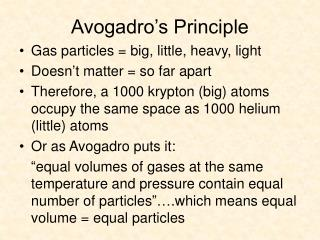# Avogadro’s Principle - PowerPoint PPT PresentationDownload PresentationDownload Presentation- - - - - - - - - - - - - - - - - - - - - - - - - - - E N D - - - - - - - - - - - - - - - - - - - - - - - - - - -
##### Presentation Transcript

1. Avogadro’s Principle • Gas particles = big, little, heavy, light • Doesn’t matter = so far apart • Therefore, a 1000 krypton (big) atoms occupy the same space as 1000 helium (little) atoms • Or as Avogadro puts it: “equal volumes of gases at the same temperature and pressure contain equal number of particles”….which means equal volume = equal particles

2. Molar Volume of Gases • The volume for one mole of gas at STP • STP = standard temperature & pressure = 0.00o C and 1.00 atm = 22.4 L for any gas (remember Avogrado) • Can be used to calculate number of particles, moles or mass of a gas.

3. Try It • How many moles of gas would be found in 34.36 L of nitrogen? How many molecules of nitrogen would this be? • Calculate the volume that .881 mol of oxygen will occupy at STP • Calculate the volume of gas for 20.00 x 1023 molecules of carbon dioxide

4. Answers • 34.36 L x 1 mole / 22.4 L = 1.534 mol • 1.534 mol x (6.02 x 1023 molecules / 1 mole) = 9.235 x 1023 molecules • .881 mol x 22.4 L / 1 mole = 19.7 L • (20.00 x 1023 molecules) x (1 mole / 6.02 x 1023 molecules) = 3.32 moles 3.32 moles x 22.4 L / 1 mole = 74.4 L

5. Try It • Calculate the volume that 4.5 kg of ethylene gas (C2H4) will occupy at STP. • How many grams of methane are (CH4) in 3,000 L?

6. Answers • 4.5 kg x 1000 g / 1 kg = 4500 g C2H4 4500 g x 1 mole / 28.05 g = 160.4 mol 160.4 mol x 22.4 L / 1 mol = 3, 593 L • 3,000 L CH4 x 1 mole / 22.4 L = 134 mol 134 mol x 16.04 g / 1 mol = 2150 g or 2.15 kg

7. Ideal Gases • Gases whose particles take up no space and have no intermolecular interactions, regardless of the pressure and temperature of the gas. • No such thing as an ideal gas since all gases have some volume & some intermolecular force

8. Ideal Gas Deviations • Real gases behave very close to what the ideal gas law predicts • Except for two extreme conditions: really high pressure or really low temperature • And 2 types of molecules: polar and large molecules

9. Ideal Gas Law • Includes moles or number of gas particles in the combined gas law • An increase in moles or particles leads to a change in at least one of the other 3 variables (temperature, pressure, volume) • So PV / T = constant that depends on the number of moles of gas involved thus….

10. Ideal Gas Law • Ideal Gas Law: PV = nRT or PV = mRT/M n = # of moles R = gas constant • Value for R depends on unit used for pressure (if atm, R = .0821 L.atm / mol.K) • n can also = m / M m = mass present M = molar mass

11. Try It • Calculate the number of moles of gas contained in a 3.0 L vessel at 300 K with a pressure of 1.50 atm. • Determine the kelvin temperature required for .0470 mol of gas to fill a balloon to 1.20 L under .988 atm pressure.

12. Answers • PV = nRT so n = PV / RT 1.50 atm x 3.0 L / .0821 L.atm / mol.K x 300 K = .18 mol • PV = nRT so T = PV / nR .988 atm x 1.20 L / .0470 mol x .0821 L.atm / mol.K = 307 K or 34o C

13. Density & Ideal Gases • Since PV = mRT / M where m = mass present M = molar mass • Then M = mRT /PV or M = DRT/P where D = density • This means the ideal gas law can be used to calculate a gas’ density

14. Try It • What is the molar mass of a pure gas that has a density of 1.40 g/L at STP? • Calculate the density a gas will have at STP if it molar mass is 44.09 g/mol.

15. Answers • M = DRT / P = 1.40g/L x .0821 L.atm / mol.K x 273 K / 1.00 atm = 31.4 g/mol • D = MP / RT = 44.09 g/mol x 1.00atm / .0821L.atm / mol.K x 273K = 1.97 g/L or .00197 g/ml